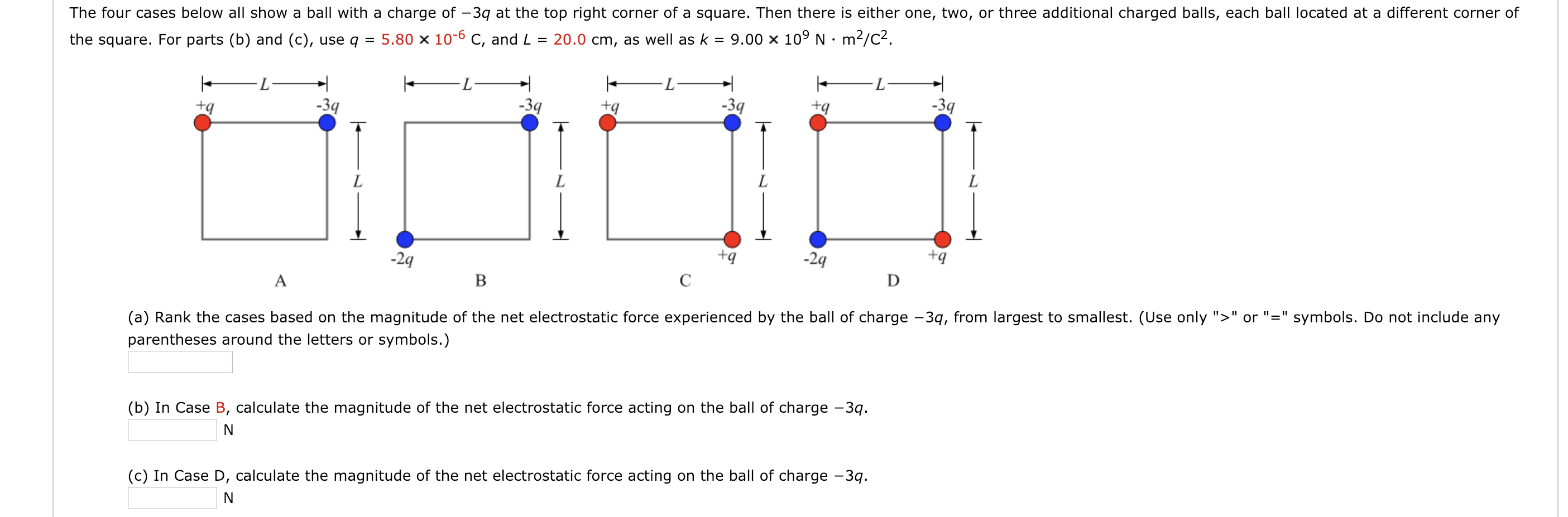# The four cases below all show a ball with a charge of -3q at the top right corner of a square. Then there is either one, two, or three additional charged balls, each ball located at a different corner ofthe square. For parts (b) and (c), use q 5.80 x 10-6 C, and L 20.0 cm, as well as k9.00 x 109 N m2/c22q-2qtq(a) Rank the cases based on the magnitude of the net electrostatic force experienced by the ball of charge -3q, from largest to smallest. (Use only "orsymbols. Do not include anyparentheses around the letters or symbols.)(b) In Case B, calculate the magnitude of the net electrostatic force acting on the ball of charge -3qIae D, calculate the magnitude of the net electrostatic force acting on the ball of charge -3

Question
86 viewshelp_outlineImage TranscriptioncloseThe four cases below all show a ball with a charge of -3q at the top right corner of a square. Then there is either one, two, or three additional charged balls, each ball located at a different corner of the square. For parts (b) and (c), use q 5.80 x 10-6 C, and L 20.0 cm, as well as k9.00 x 109 N m2/c2 2q -2q tq (a) Rank the cases based on the magnitude of the net electrostatic force experienced by the ball of charge -3q, from largest to smallest. (Use only "orsymbols. Do not include any parentheses around the letters or symbols.) (b) In Case B, calculate the magnitude of the net electrostatic force acting on the ball of charge -3q Iae D, calculate the magnitude of the net electrostatic force acting on the ball of charge -3 fullscreen
check_circle

Step 1

(a)

Magnitude of electristatic force FA on -3q charge in case A as given by Coloumb’s law is

Step 2

Magnitude of electristatic force FB on -3q charge in case B as given by Coloumb’s law is

Step 3

Magnitude of electristatic force FC on -3q charge in case C...

### Want to see the full answer?

See Solution

#### Want to see this answer and more?

Solutions are written by subject experts who are available 24/7. Questions are typically answered within 1 hour.*

See Solution
*Response times may vary by subject and question.
Tagged in
SciencePhysics

### Electric Charges and Fields